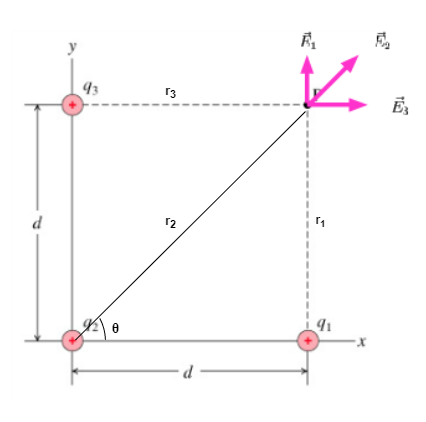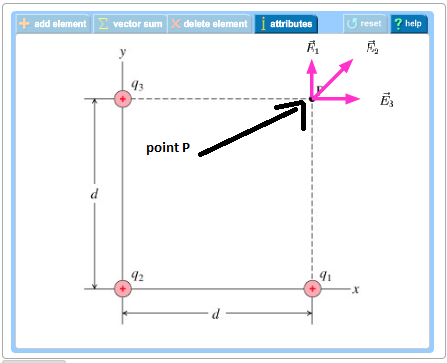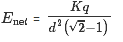# Problem: Intuitively, which of the following would happen to Enet if d became very large?A. E net should reduce to the field of a point charge of magnitude q.B. E net should reduce to the field of a point charge of magnitude 4q.C. The larger d becomes, the smaller the magnitude of E net will be.D. The larger d becomes, the greater the magnitude of E net will be.

###### FREE Expert Solution

Electric field:

$\overline{){\mathbf{E}}{\mathbf{=}}\frac{\mathbf{k}\mathbf{q}}{{\mathbf{r}}^{\mathbf{2}}}}$, where k is Coulomb constant, q is a charge, and r is the distance between the charge to the point where the electric field is to be calculated.

Let's visualize our problem in the figure below:We need to note that:

E1 = E2sinθ

93% (230 ratings)###### Problem Details

Intuitively, which of the following would happen to Enet if d became very large?

A. E net should reduce to the field of a point charge of magnitude q.

B. E net should reduce to the field of a point charge of magnitude 4q.

C. The larger d becomes, the smaller the magnitude of E net will be.

D. The larger d becomes, the greater the magnitude of E net will be.